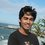# Bangladesh National Math Olympiad 2014 : Junior level Problem 10

Oindri has$$100$$ chocolates. She finished eating all her chocolates in $$58$$ days by eating at least one chocolate each day. Prove that, in some consecutive days, she eats exactly $$15$$ chocolates.

(It was probably the hardest problem for the Junior level this year. Hope you guys have fun solving it.)

[SPOILER!!]

[SPOILER!!]

[SPOILER!!]

In case you did not get the statement, here's a better explanation. Define $d_i =$ the number of chocolates she ate on the $i^{th}$ day. You have to prove that, for some $i$ and $j$, $\sum_{k=i}^j d_k = 15$ where $1\leq i\leq j\leq 58$.Note by Labib Rashid
7 years, 3 months ago

This discussion board is a place to discuss our Daily Challenges and the math and science related to those challenges. Explanations are more than just a solution — they should explain the steps and thinking strategies that you used to obtain the solution. Comments should further the discussion of math and science.

When posting on Brilliant:

• Use the emojis to react to an explanation, whether you're congratulating a job well done , or just really confused .
• Ask specific questions about the challenge or the steps in somebody's explanation. Well-posed questions can add a lot to the discussion, but posting "I don't understand!" doesn't help anyone.
• Try to contribute something new to the discussion, whether it is an extension, generalization or other idea related to the challenge.
• Stay on topic — we're all here to learn more about math and science, not to hear about your favorite get-rich-quick scheme or current world events.

MarkdownAppears as
*italics* or _italics_ italics
**bold** or __bold__ bold
- bulleted- list
• bulleted
• list
1. numbered2. list
1. numbered
2. list
Note: you must add a full line of space before and after lists for them to show up correctly
paragraph 1paragraph 2

paragraph 1

paragraph 2

[example link](https://brilliant.org)example link
> This is a quote
This is a quote
    # I indented these lines
# 4 spaces, and now they show
# up as a code block.

print "hello world"
# I indented these lines
# 4 spaces, and now they show
# up as a code block.

print "hello world"
MathAppears as
Remember to wrap math in $$ ... $$ or $ ... $ to ensure proper formatting.
2 \times 3 $2 \times 3$
2^{34} $2^{34}$
a_{i-1} $a_{i-1}$
\frac{2}{3} $\frac{2}{3}$
\sqrt{2} $\sqrt{2}$
\sum_{i=1}^3 $\sum_{i=1}^3$
\sin \theta $\sin \theta$
\boxed{123} $\boxed{123}$

## Comments

Sort by:

Top Newest

Let $f(n)$ be the number of chocolates eaten by the end of day n. There are only 15 possible remainders $\pmod{15}$ so by the pigeonhole principle we have 2 cases: The first case is that there at least 13 sets of 4 which satisfy the following congruence: $f(a) \equiv f(b) \equiv f(c) \equiv f(d) \pmod{15}$ where $f(d) > f(c) > f(b) > f(a)$. Also $f(d)-f(c) \equiv f(c)-f(b) \equiv f(b)-f(a) \equiv 0 \pmod {15}$. Say that there was no set of consecutive days where Oindri ate 15 chocolates. Then we know that $f(d)-f(c), f(c)-f(b), f(b)-f(a) \ge 30$. Therefore $f(d)-f(a) \ge 90$. Since she only has 100 chocolates we know in fact that $f(d)-f(a) = 90$. But we have this fact 13 times over, ie. $f(d_{1})-f(a_{1}) = f(d_{2})-f(a_{2}) = ... = f(d_{13})-f(a_{13}) = 90$. But all $f(a_{k})$ are distinct, so $f(a_{i}) \ge 13$ for some value of i where $1 \leq i \leq 13$. But that implies that $f(d_{i}) \ge 103$ which is more than the number of chocolates, so we have a contradiction. Therefore our assumption was wrong and there must be some set of consecutive days where Oindri ate 15 chocolates. The second case is that there is one set of at least 5 terms which satisfies the following congruence $f(a) \equiv f(b) \equiv f(c) \equiv f(d) \equiv f(e) \pmod{15}$ where $f(e) > f(d) > f(c) > f(b) > f(a)$. Using just the same assumption and argument as before we realise that $f(e) \ge f(a) + 120$, but this again will be more than the number of chocolates, again meaning that our assumption was wrong and there must be some set of consecutive days where Oindri ate 15 chocolates.

- 7 years, 3 months ago

Log in to reply

what is pigeonhole priniciple?

- 7 years, 1 month ago

Log in to reply

It's like when you have more pigeons than holes, you need more holes.

- 4 years, 4 months ago

Log in to reply

thanks for this invaluable information :3

- 4 years, 4 months ago

Log in to reply

could u pls solve it more simply?

- 5 years, 7 months ago

Log in to reply

I was unable to answer this question at all :(

- 7 years, 1 month ago

Log in to reply

×

Problem Loading...

Note Loading...

Set Loading...